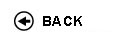Politecnico di Torino
Academic Year 2017/18
02OGGLM
Signal analysis and processing
1st degree and Bachelor-level of the Bologna process in Computer Engineering - Torino
 Teacher Status SSD Les Ex Lab Tut Years teaching Poggiolini PierluigiPO ING-INF/03 80 20 0 0 8
 SSD CFU Activities Area context ING-INF/03 8 B - Caratterizzanti Ingegneria delle telecomunicazioni
 Subject fundamentals The class describes the main fundamental analysis and processing techniques for deterministic and random continuous-time signals (first part), and for deterministic and random discrete-time signals (second part). The topics are quite multidisciplinary in the sense that these notions and techniques are used in many of the classes that follow. Expected learning outcomes The specific knowledge and abilities that the student will acquire are: - Knowledge of the classification of signals. Knowledge of frequency analysis for continuous-time signals. Knowledge of linear time-invariant (LTI) systems, as well as of their representation in the time and frequency domains. Knowledge of the basic types of signal filters. Knowledge of random signals (called random processes), of their statistical characterization and of their spectral representation. - Ability to classify signals with respect to their properties. Ability to transform and analyze a signal in the time and frequency domains. Ability to classify and analyze a LTI system in the time and frequency domains. Ability to statistically describe a random process and to characterize its spectral properties, as well as its interactions with LTI systems. - Knowledge of the techniques for passing from a continuous-time to discrete-time signal, and vice-versa. Knowledge of the techniques for digital processing of a signal in the frequency domain. Knowledge of the techniques for discrete-time processing of digital signals in the frequency domain. Knowledge of the techniques for analysis of LTI systems in discrete-time, and of the Z-transform. Knowledge of digital filters structures (FIR, IIR), and their design techniques. - Ability to pass from discrete time to continuous time signals, and vice-versa. Ability to process signals and systems in the time and frequency domain. Ability to analyze and design discrete-time LTI systems. The ability to apply the gained knowledge will be verified through problems assigned in class, as well as during the written and oral final examination. The oral examination will also help students improve their communication skills. Prerequisites / Assumed knowledge Fundamental notions of linear algebra, Euclidean spaces and the representation of their elements in terms of components vs. a basis. Complex analysis of functions in one or two variables. Probability theory: discrete and continuous random variables, probability density function, expectation operator. Fourier series, Fourier and Laplace transforms. First order linear differential equations. Contents Topics dealt with in the class: - Signal classification; energy and power (5 hours) - Linear and inner-product spaces, signal spaces, signal canonical representation and approximants (8 hours) - Fourier series and transform (10 hours) - Linear Time Invariant (LTI) systems, impulse response and transfer function (10 hours) - Energy spectrum and autocorrelation function. Periodic signals and power spectral density (10 hours) - Random processes (20 hours) - Sampling theorem (5 hours) - Discrete time signals: basic operations, energy and power (4 hours) - Discrete time Fourier transform, circular convolution, discrete time Fourier transform (10 hours) - Discrete time LTI systems: time and frequency analysis, Z transform based analysis (8 hours) - Digital filters with finite (FIR) and infinite (IIR) impulse response. Window-based design of FIR filters. Bilinear transformation-based design of IIR filters. (10 hours) (Hours are indicative � variations are possible.) Delivery modes Theoretical topics are dealt with in regular lectures. Regarding problem-solving, either the teacher solves problems in class on the topics introduced during the lecture, or the students work independently on the suggested problems with guidance from the teacher. Texts, readings, handouts and other learning resources Textbooks: 1. P. Poggiolini and M. Visintin, Class Notes on Signal Analysis and Processing (downloadable from the course portal). For further (optional) reading: 2. Luca Mesin, Introduction to signal theory, CLUT. 3. L. Lo Presti e F. Neri, L'analisi dei segnali, CLUT, 1992. 4. L. Lo Presti e F. Neri, Elaborazione numerica dei segnali, Pearson, 2007. 5. M. Laddomada e M. Mondin, Introduzione ai processi casuali, CLUT, 1992. 6. A. Papoulis e S. U. Pillai, Probability, Random Variables and Stochastic Processes, McGraw-Hill, 2002. Assessment and grading criteria The final exam is both written and oral. The student is asked to solve at least three problems, which he/she has to solve in writing. However, the process is interactive with the teachers, who ask questions about the methods used to solve the problem and may ask additional theoretical questions related to the problems. The exam lasts about 1.5 to 2 hours on average. The three questions deal with the three main sections of the class: deterministic time-continuous signals and linear systems, discrete-time signals and linear systems, random processes (one each). The overall exam is "closed books". Programma definitivo per l'A.A.2017/18© Politecnico di Torino
Corso Duca degli Abruzzi, 24 - 10129 Torino, ITALY